# Lions Weekly: October 6, 2021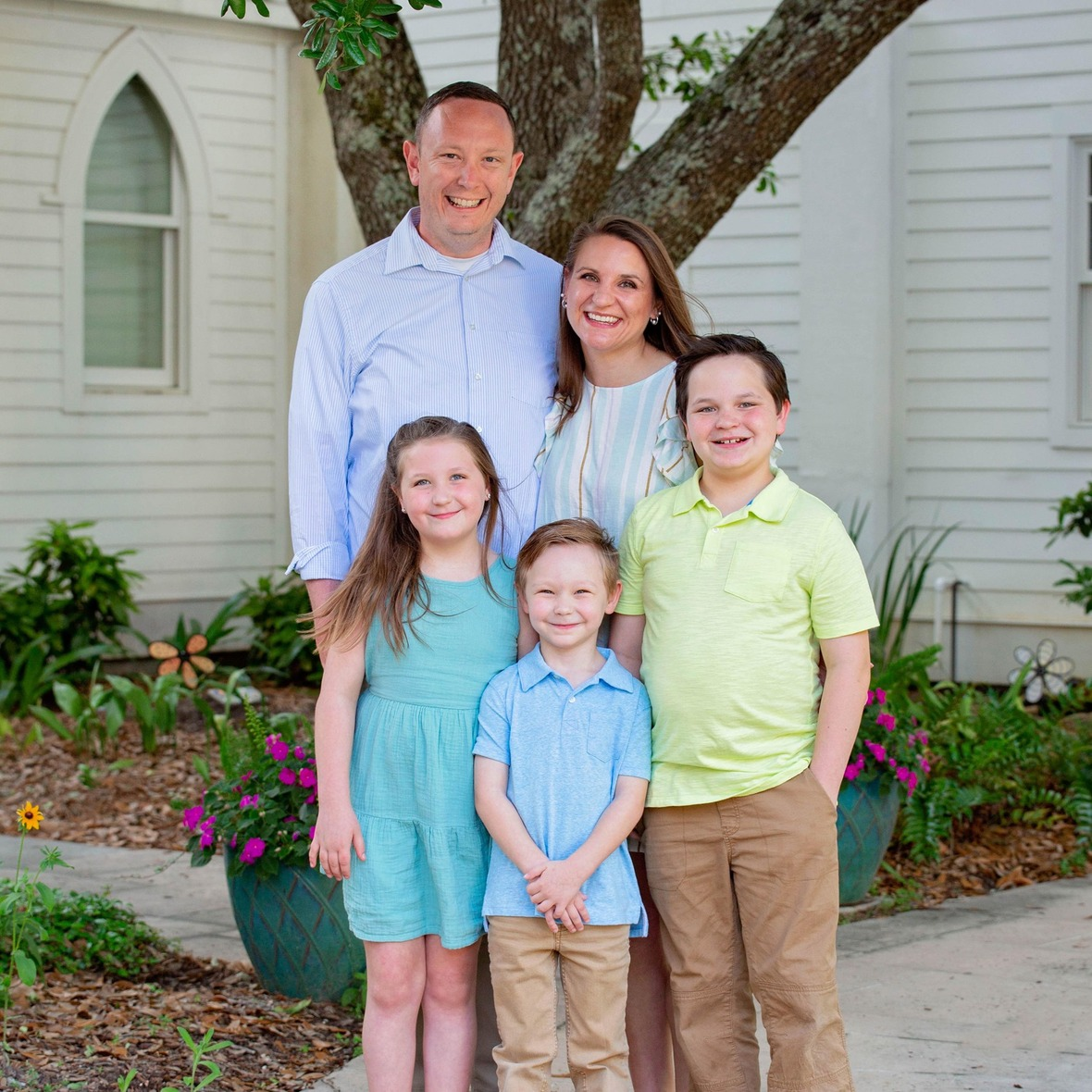# A Note From Mr. Sandefur

Lion Family,

Good afternoon! While I cherish my opportunity to address our school community on a weekly basis, I rarely use the opportunity for how I must do so today. As a leader of this institution, my desire is to effectively communicate with our school community so that we are all on the same page and working towards the same goal. As an educator, my heart is first and foremost for our students to experience the love of Christ in all that occurs in their educational journey. Unfortunately, the world that we live in is at most times at odds with that goal.

Many of you may be aware, however, I felt it necessary to bring a recent cultural trend to our school community’s attention so that we can partner together effectively. A social media trend has taken our nation’s schools by storm recently as students are encouraged to commit “devious” acts on their school campuses for fleeting social media “fame.” As you can imagine, the professional duties of our educators are already expansive without the addition of such behavior. I am asking that our families (middle and high school) have conversations with their students about their behavior choices and making sure they understand our stance as a school that such acts cannot and will not be tolerated. It is my hope that through our partnership, students will see the paths of this world and the path of Christ are separate. As followers of Christ, our behavior cannot emulate the behavior of the world. I truly appreciate your support in this endeavor.

I pray that the upcoming break is a time of rest and rejuvenation for you and your family. I would like to request your prayers as I embark to Kenya on behalf of TKA. We are looking to develop a partnership with a local school there, beginning with a pen pal program to enrich the educational process for our students as well as theirs. This trip will also lay the groundwork for future trips for members of our school community to broaden our worldview as well as to impact the world for Christ.

In Christ Alone,

Jeremy Sandefur
President

 table div table+table+table+table div table{width:100%;padding:0}table div table+table+table+table div table img{width:96.23%;padding:0;float:none}table div table+table+table+table div table td{width:100%;padding:0 1.88% 18px}/* styles */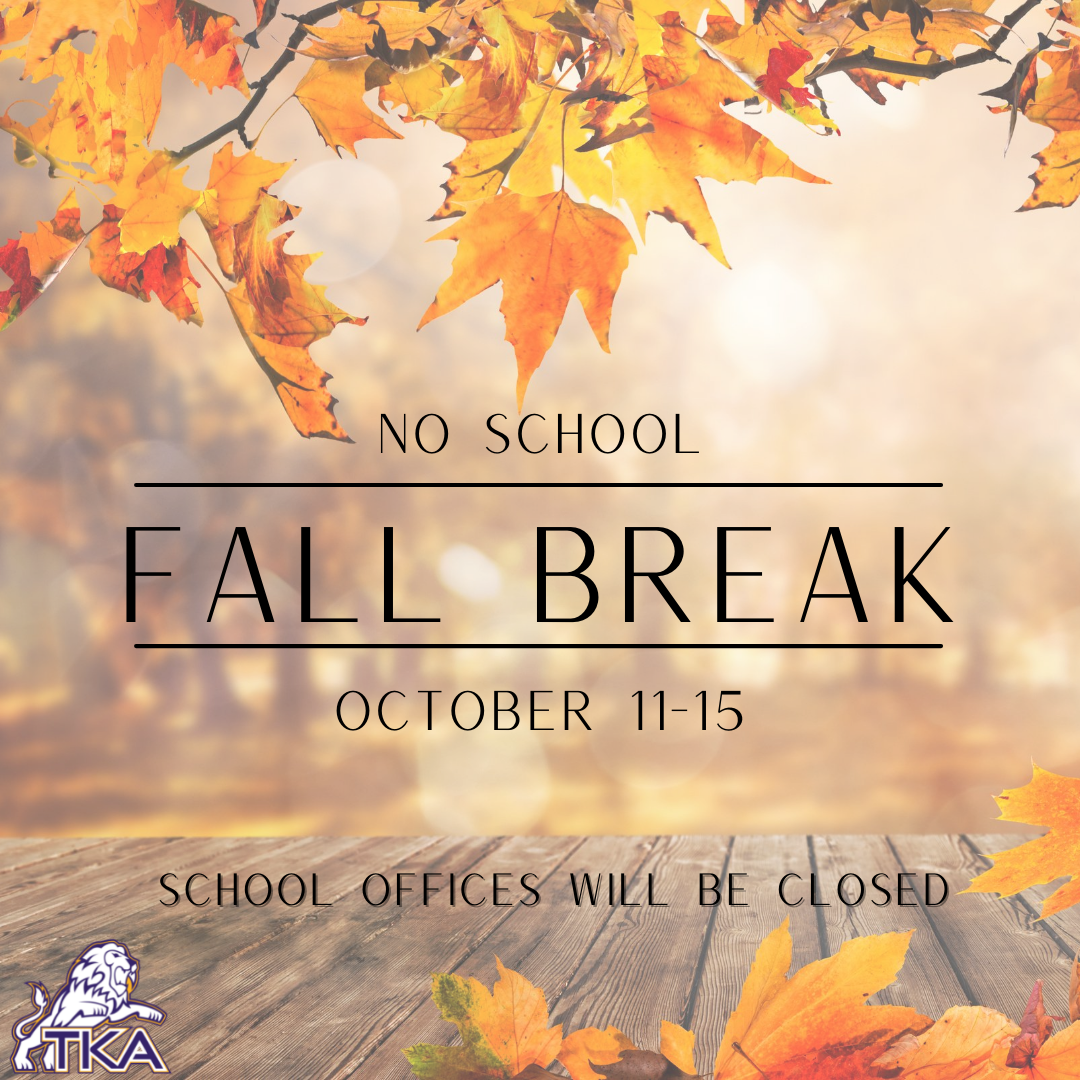# Fall Break

TKA's Fall Break will be taking place during the week of Monday, October 11- Friday, October 15. School offices will be closed during this time.

Lion's Weekly will not be sent out during fall break. It will resume on Wednesday, October 20.# Teacher Work Days

Monday, October 18 - Tuesday, October 19 are teacher work days. No school will take place during these days.# Tuesday, October 19

PreK3- 12 grade parent conferences will be taking place during this day. Preschool and elementary parents need to sign up for a conference time by contacting your student's teacher. Middle school and High school parents can sign up for conference times by clicking here.

 table div table+table+table+table+table+table+table+table div table{width:100%;padding:0}table div table+table+table+table+table+table+table+table div table img{width:96.23%;padding:0;float:none}table div table+table+table+table+table+table+table+table div table td{width:100%;padding:0 1.88% 18px}/* styles */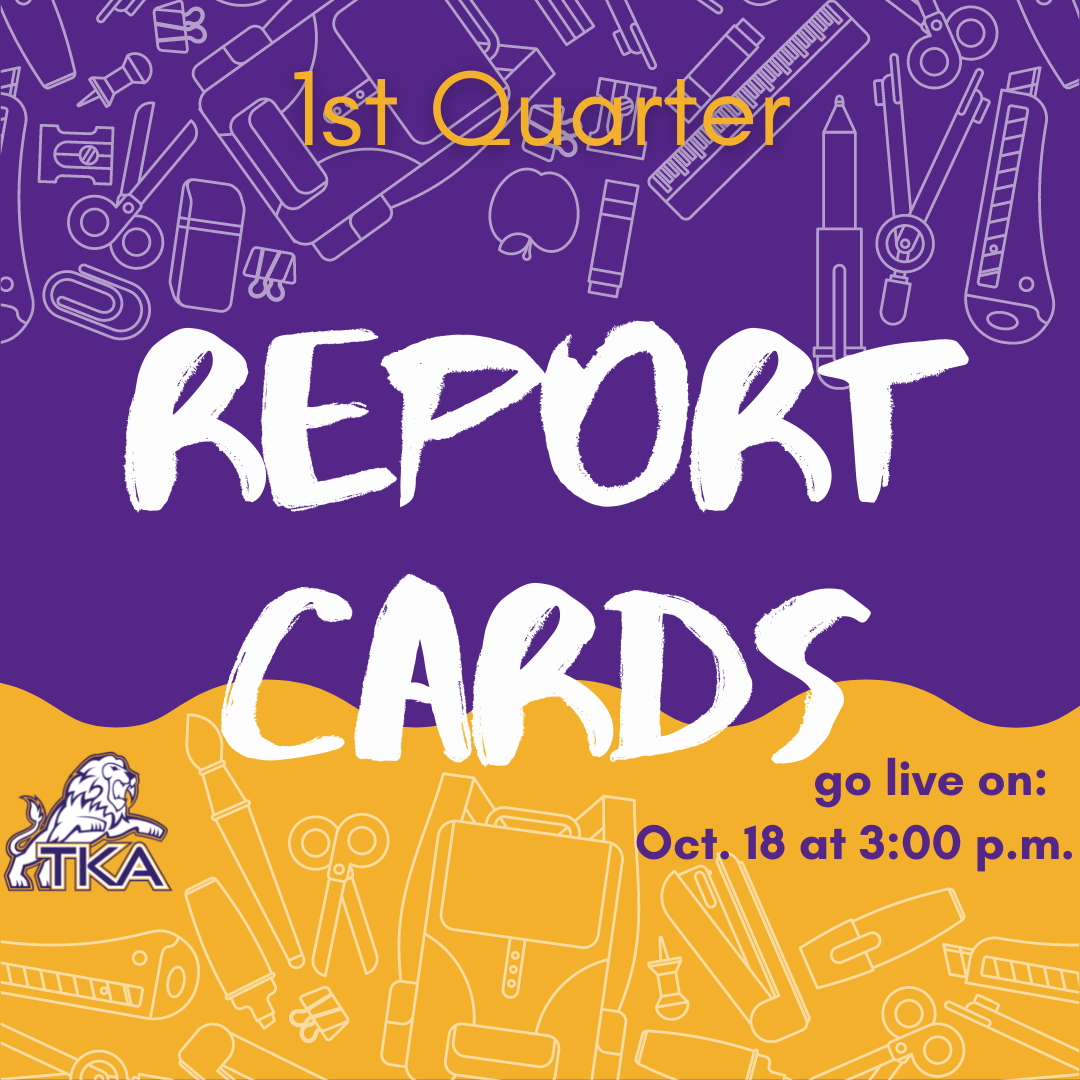# Report Cards

First quarter report cards will go live on the FACTS family portal, Monday, October 18, at 3:00 p.m.

 table div table+table+table+table+table+table+table+table+table+table div table{width:100%;padding:0}table div table+table+table+table+table+table+table+table+table+table div table img{width:96.23%;padding:0;float:none}table div table+table+table+table+table+table+table+table+table+table div table td{width:100%;padding:0 1.88% 18px}/* styles */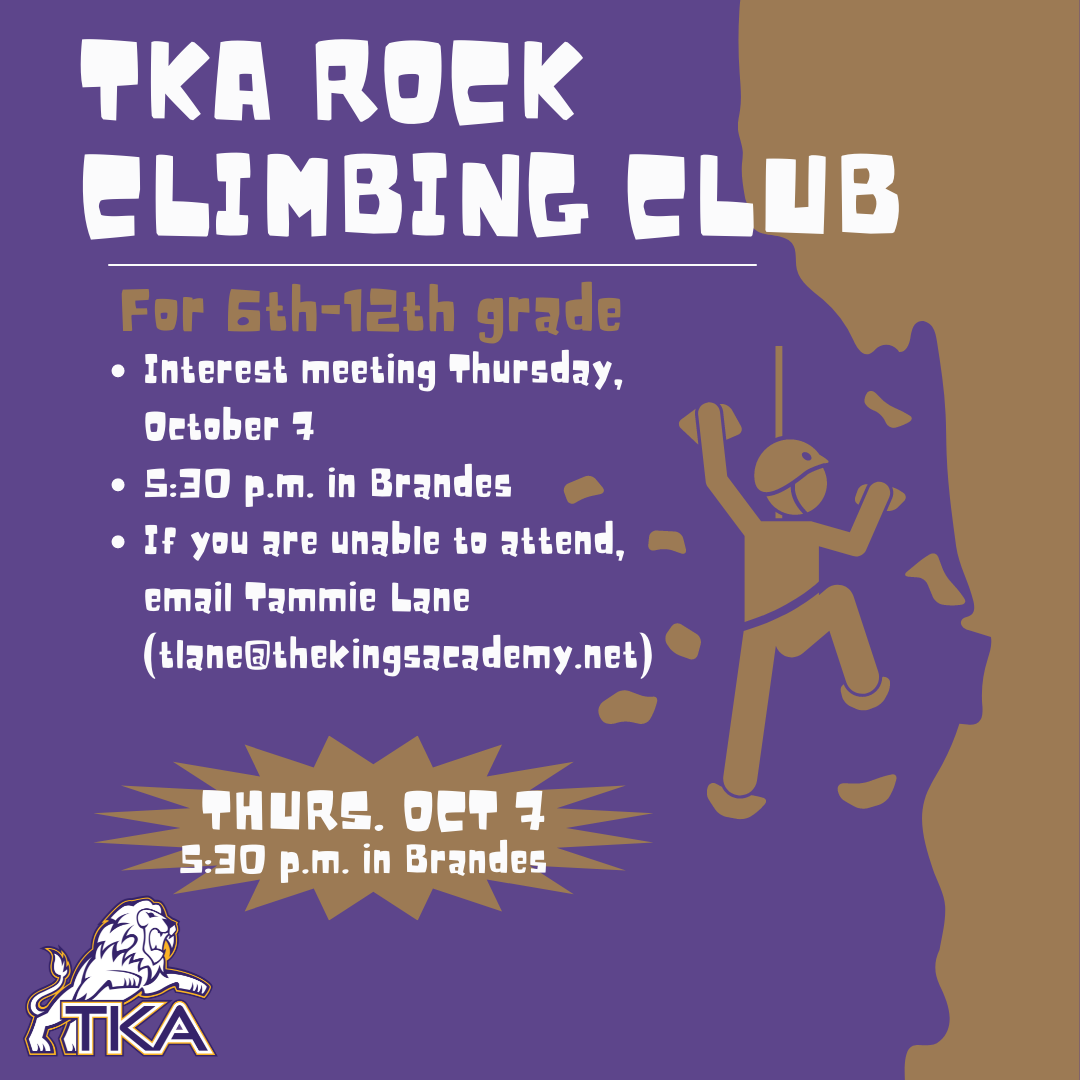# TKA Rock Climbing Club

We would like to invite all 6th - 12th graders interested in participating in the Rock Climbing Club to an interest meeting on Thursday, October 7th at 5:30 p.m. in Brandes. Anyone interested in participating in the club who is unable to attend can contact our Rock Climbing Coach/Facilitator Tammie Lane, (tlane@thekingsacademy.net) for more information.

No prior climbing experience needed or required!

 table div table+table+table+table+table+table+table+table+table+table+table+table div table{width:100%;padding:0}table div table+table+table+table+table+table+table+table+table+table+table+table div table img{width:96.23%;padding:0;float:none}table div table+table+table+table+table+table+table+table+table+table+table+table div table td{width:100%;padding:0 1.88% 18px}/* styles */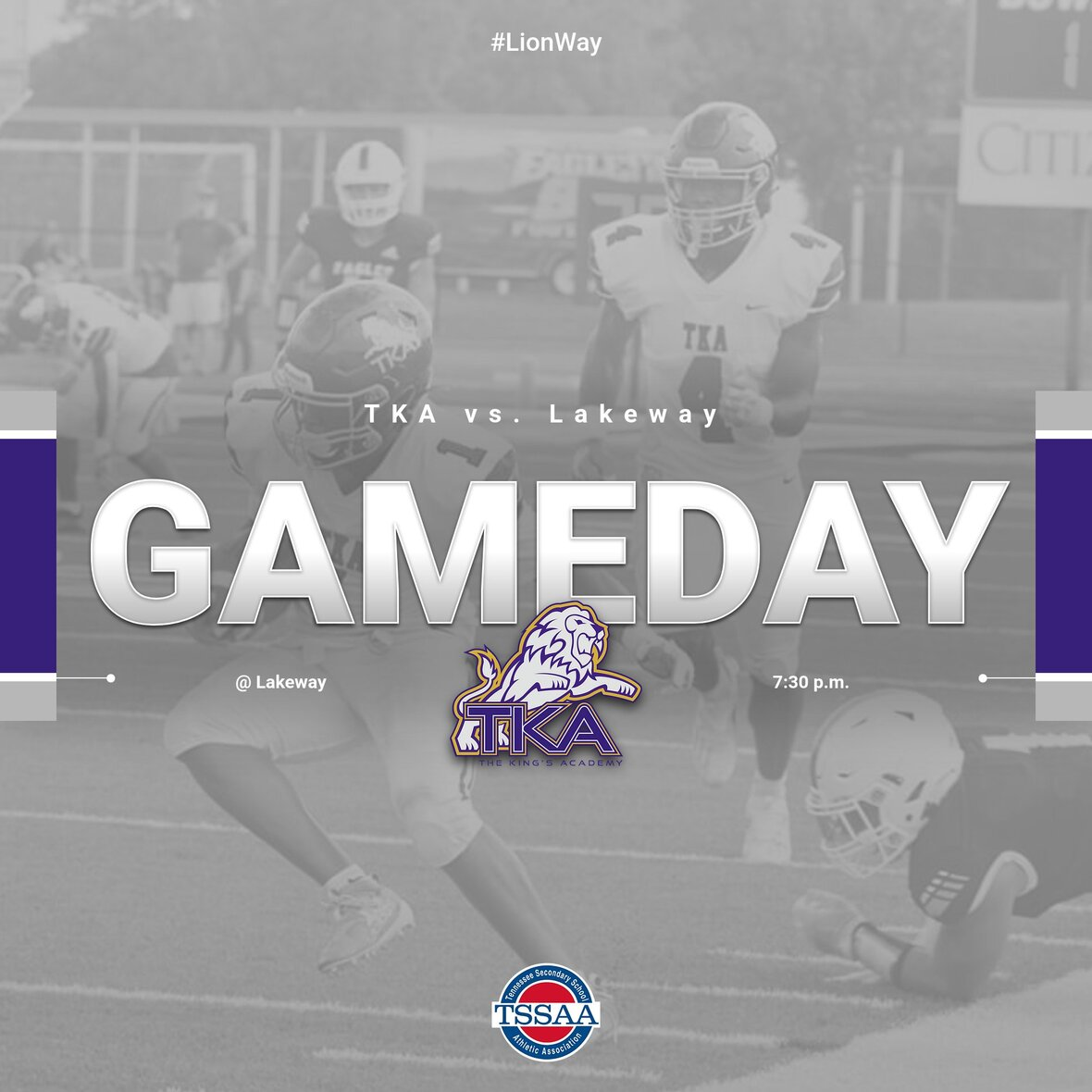# Varsity Football

TKA is looking forward to playing at Lakeway Christian this Friday, October 8, at 7:30 p.m. Tickets will be sold at the gate. We hope to see you there!

 /* styles */
 table div table+table+table+table+table+table+table+table+table+table+table+table+table+table+table div table{width:100%;padding:0}table div table+table+table+table+table+table+table+table+table+table+table+table+table+table+table div table img{width:96.23%;padding:0;float:none}table div table+table+table+table+table+table+table+table+table+table+table+table+table+table+table div table td{width:100%;padding:0 1.88% 18px}/* styles */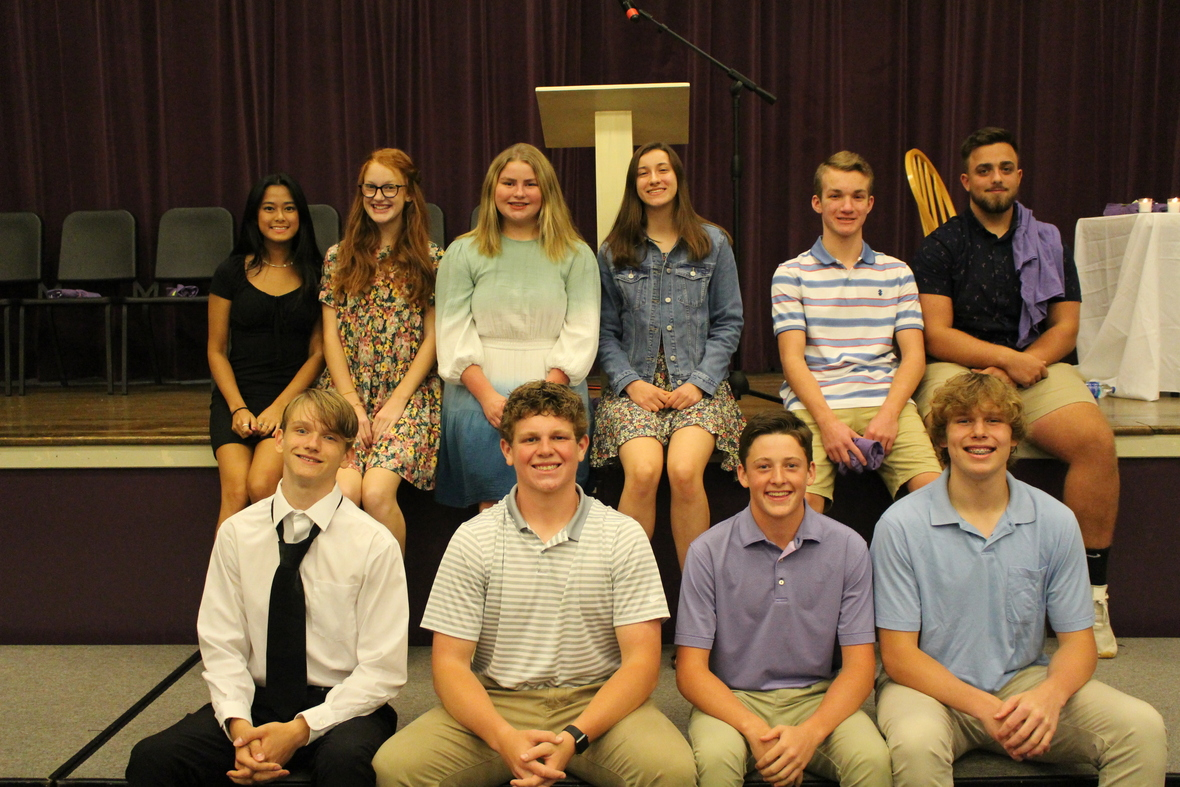# National Honors Society Inductions

Congratulations to our 2021 National Honors Society Inductees.

Seniors: Allison Dabi, Eli Knight

Sophomores: Peyton Brooks, Christian Cabeiras, Gracie Houser, Lilly Kate McKinney*, Will Rhoton, Braelyn Read, Harrison Rollins, Zac Wallace

*indicates those not pictured

 table div table+table+table+table+table+table+table+table+table+table+table+table+table+table+table+table+table div table{width:100%;padding:0}table div table+table+table+table+table+table+table+table+table+table+table+table+table+table+table+table+table div table img{width:96.23%;padding:0;float:none}table div table+table+table+table+table+table+table+table+table+table+table+table+table+table+table+table+table div table td{width:100%;padding:0 1.88% 18px}/* styles */# Pink Out Day Recap

This Friday, October 1, was pink out day! Students dressed in pink attire to support Breast Cancer Awareness.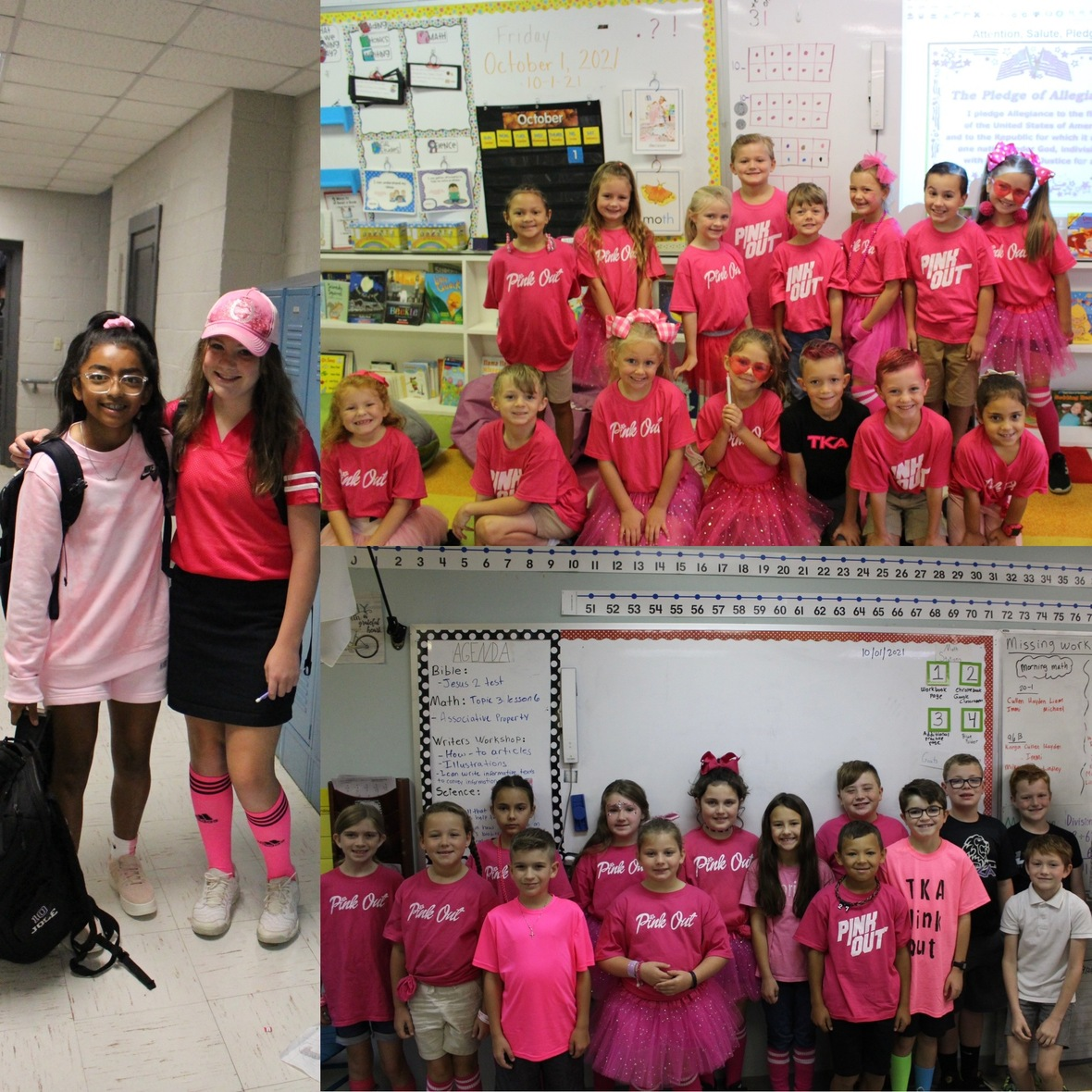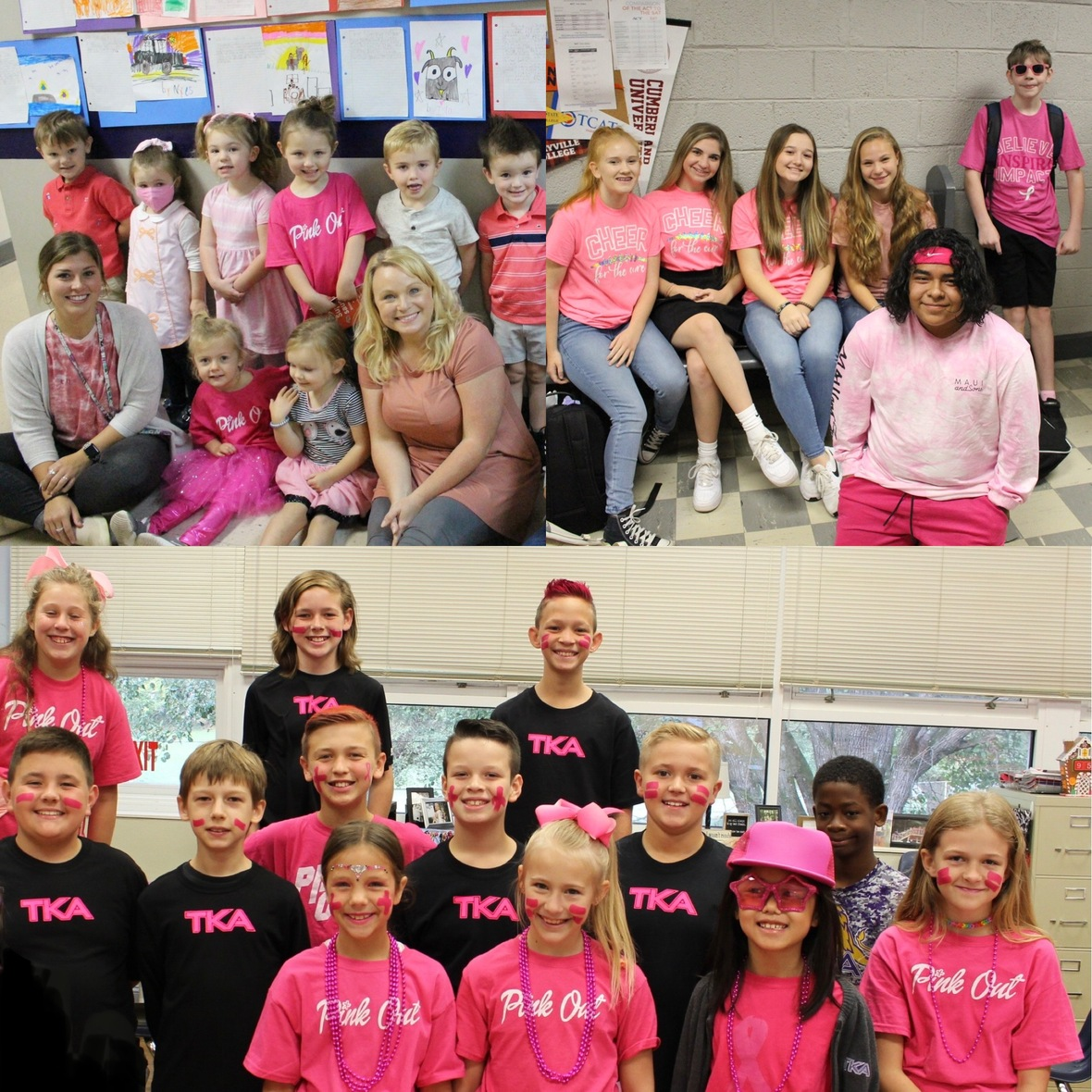# Breast Cancer Awareness

Thank you to everyone who participated in the breast cancer halftime event at the Pink Out game on Friday and the Celebration and Walk on Saturday.

During halftime and at the walk on Saturday, we recognized breast cancer survivors and honored those families who have lost a loved one due to breast cancer.

The Survivors who were recognized:
1.) Jennifer Ayers
2.) Rhonda Garbett
3.) Amanda Sutton
4.) Sharon Donato
5.) Gloria Hindenburg
6.) Ellen Longmire
7.) Barbara Mouser
8.) Elizabeth Justice
9.) Liz Wheeler
10.) Cindy Jeffers
11.) Sharon Davis
12.) Karen Coppock

The Loved ones honored who lost their battle to breast cancer:
1.) Barbara Thornton
2.) Elizabeth Jones
3.) Taunya Milender
4.) Bobbi Hamilton
5.) Jewel McVey
6.) Vivian Morrison
7.) Clara Patrick
8.) Lina Leonard
9.) Lynda Abernathy
10.) Christy Bowlin
11.) Judy *
12.) Ashley *
13.) Wendy *
14.) Trina *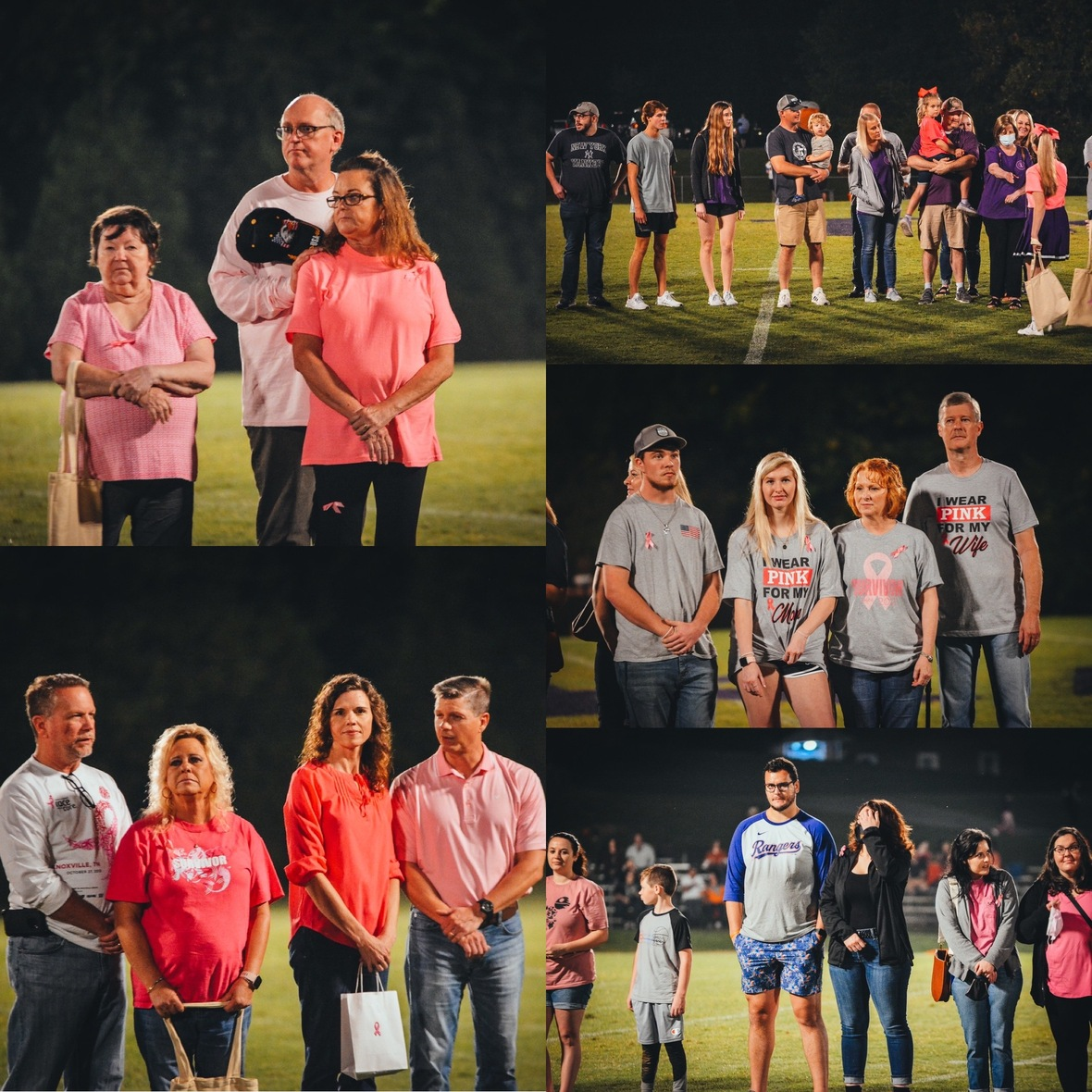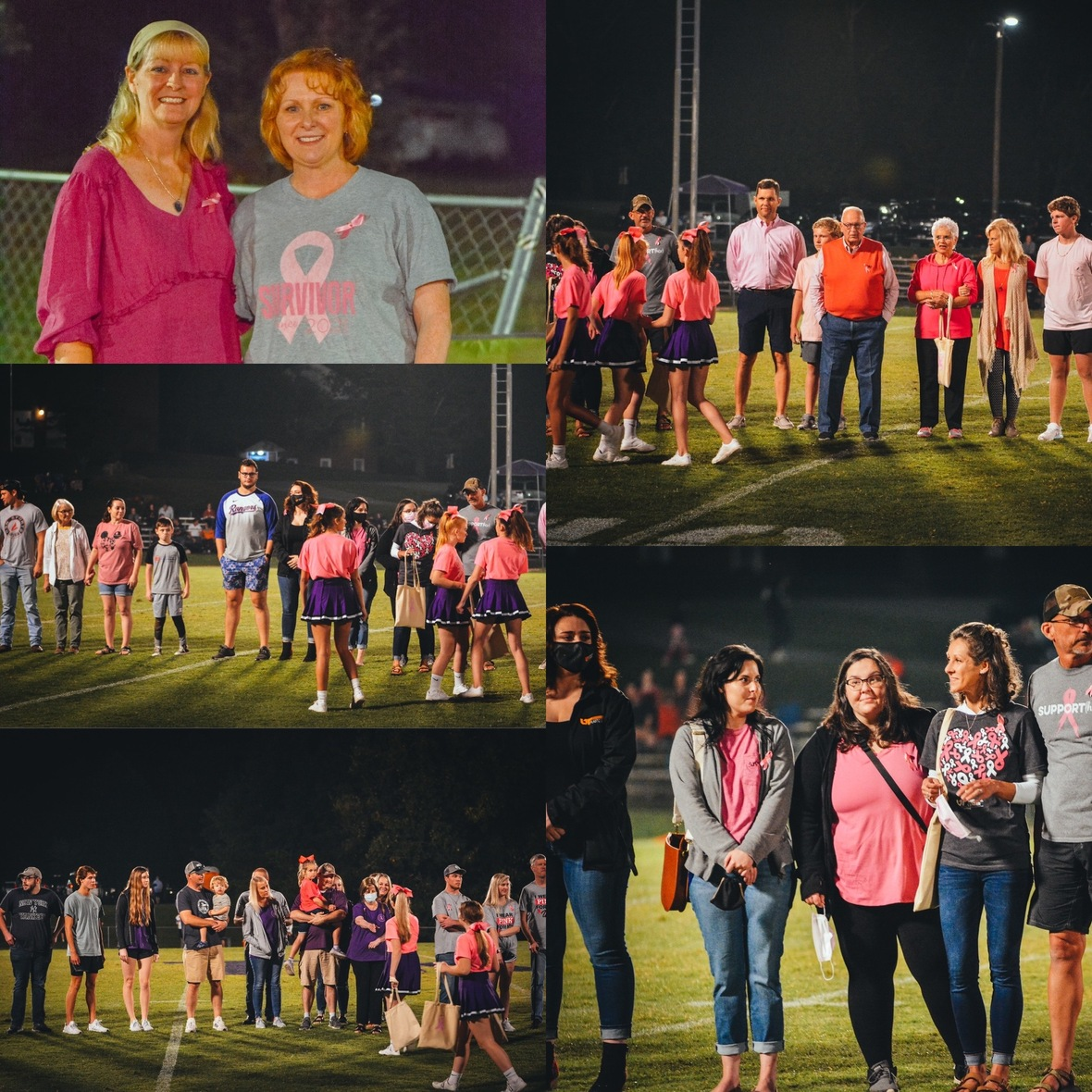# Breast Cancer Awareness Walk

We were thrilled to raise \$1,055 to go towards a patient at UT Hospital (through the Stephens Cancer Care Fund) who is currently going through breast cancer treatments. Saturday was an amazing day and we were so thankful for everyone's generosity and support. We look forward to having this event again next year!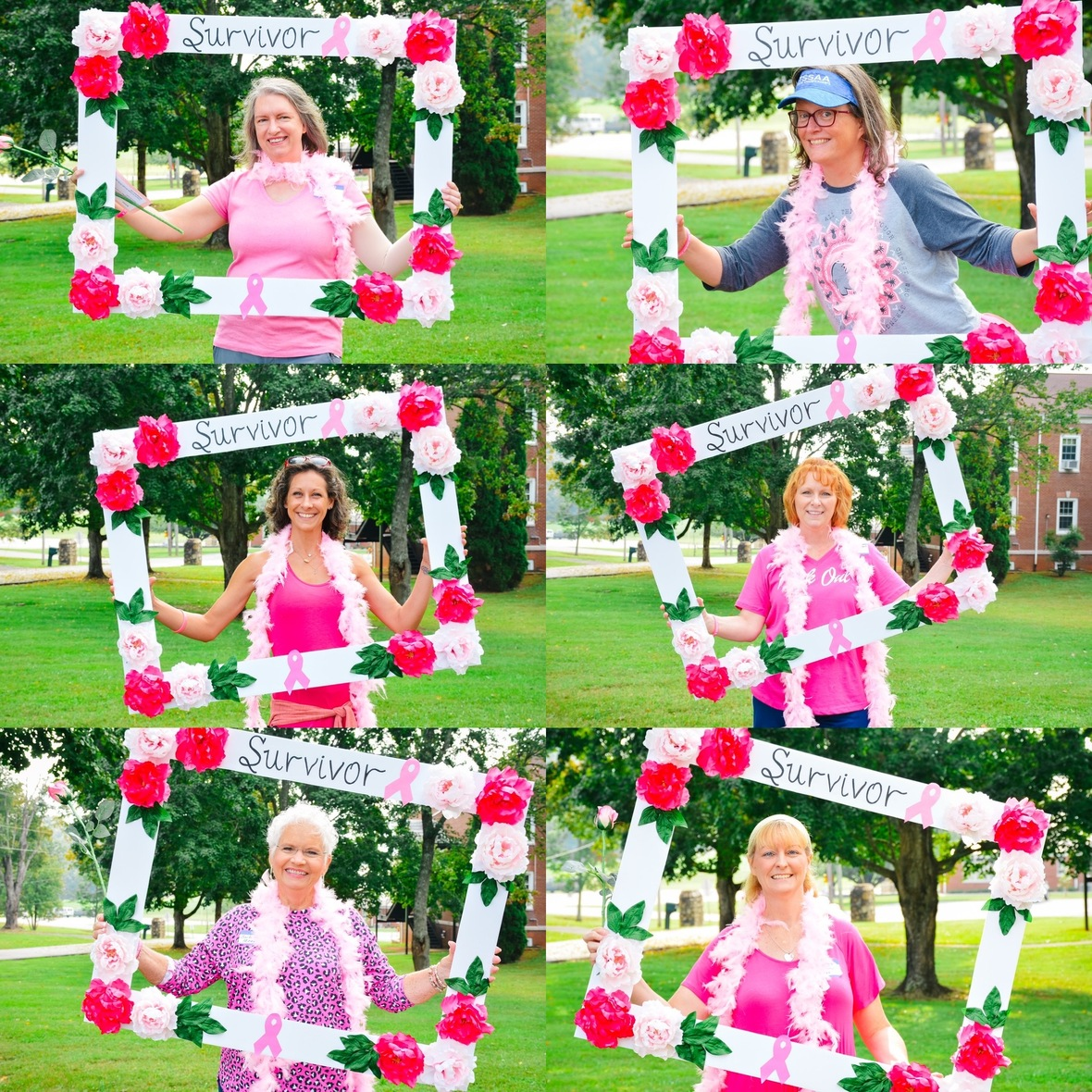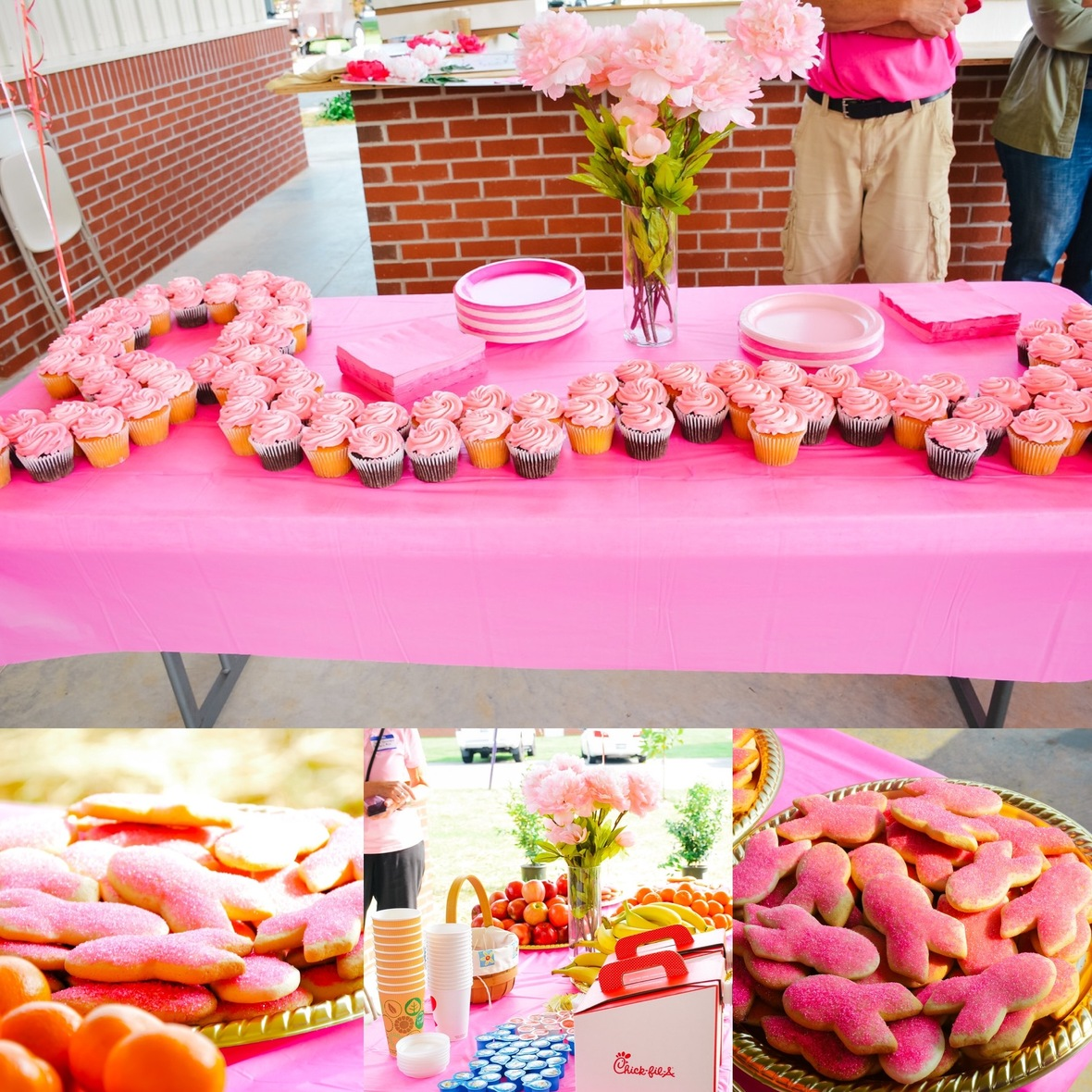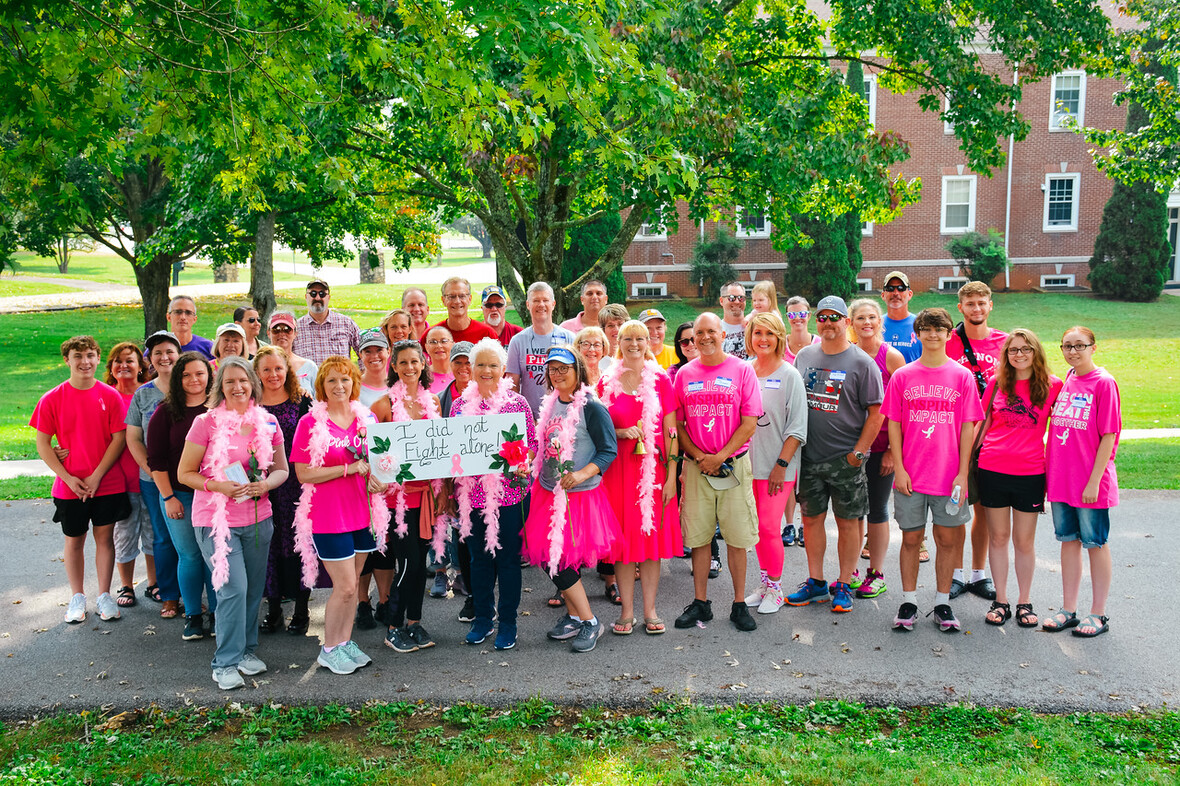table div table+table+table+table+table+table+table+table+table+table+table+table+table+table+table+table+table+table+table+table+table+table+table+table+table+table+table+table+table+table div table{width:100%;padding:0}table div table+table+table+table+table+table+table+table+table+table+table+table+table+table+table+table+table+table+table+table+table+table+table+table+table+table+table+table+table+table div table img{width:96.23%;padding:0;float:none}table div table+table+table+table+table+table+table+table+table+table+table+table+table+table+table+table+table+table+table+table+table+table+table+table+table+table+table+table+table+table div table td{width:100%;padding:0 1.88% 18px}/* styles */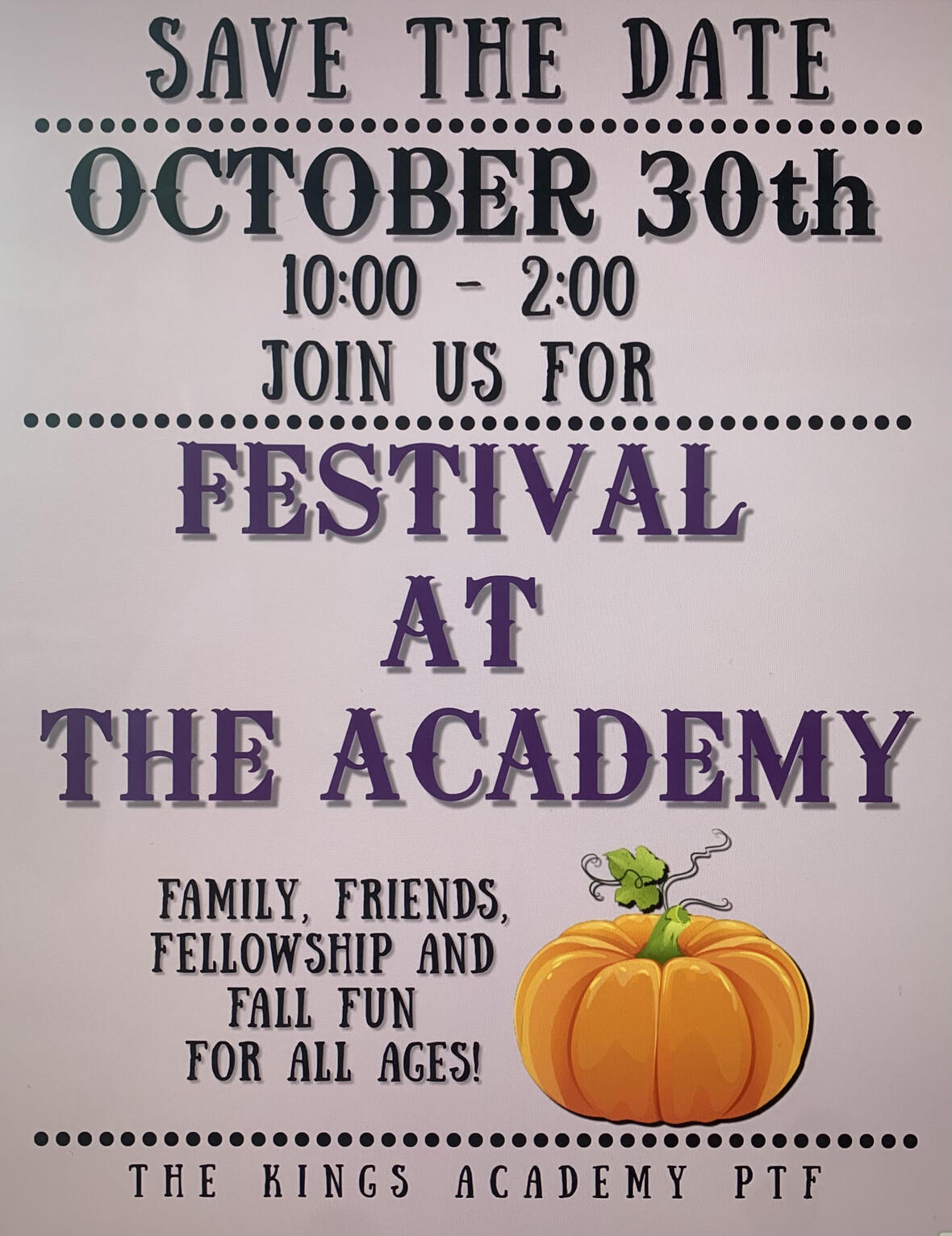Mark your calendars and save the date! The Festival at the Academy is taking place on Saturday, October 30, from 10 a.m. to 2 p.m.

The committee is currently taking donations for the silent auction baskets. If you or your company would like to donate a tax deductible basket, reach out to one of the contacts listed below!

## Contacts for Festival at The Academy:

The King’s Academy (865) 573-8321 ext.5
Kara Pritchard (865) 924-4333
Cherish McLin (865) 604-8303

 table div table+table+table+table+table+table+table+table+table+table+table+table+table+table+table+table+table+table+table+table+table+table+table+table+table+table+table+table+table+table+table+table div table{width:100%;padding:0}table div table+table+table+table+table+table+table+table+table+table+table+table+table+table+table+table+table+table+table+table+table+table+table+table+table+table+table+table+table+table+table+table div table img{width:96.23%;padding:0;float:none}table div table+table+table+table+table+table+table+table+table+table+table+table+table+table+table+table+table+table+table+table+table+table+table+table+table+table+table+table+table+table+table+table div table td{width:100%;padding:0 1.88% 18px}/* styles */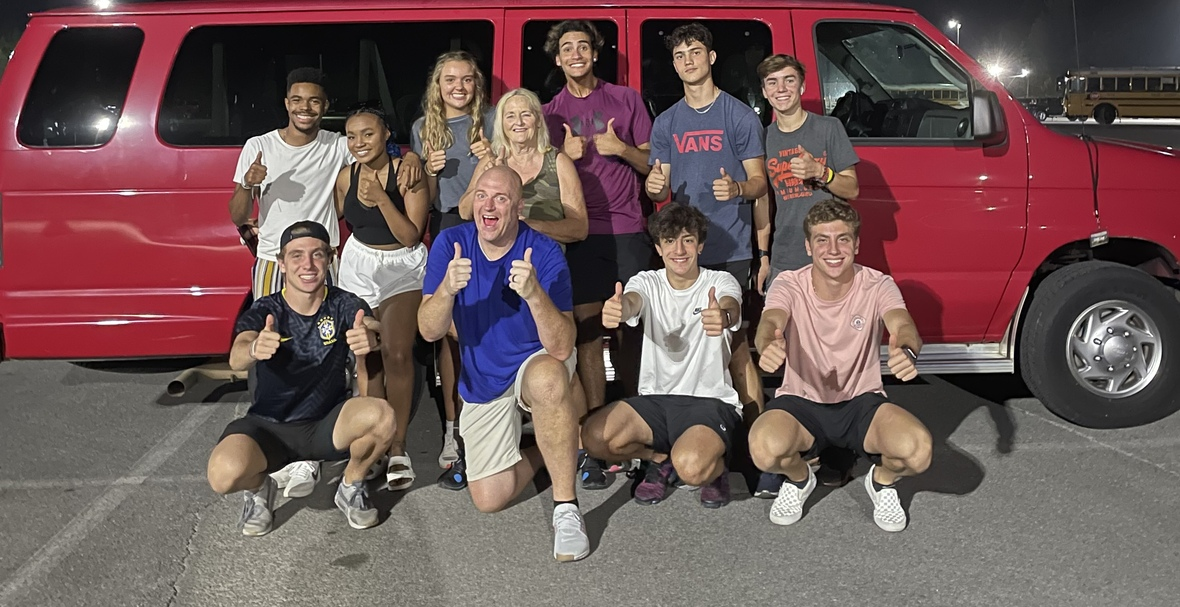# Residence Life in Action

This weekend, our residence life students spent their Saturday exploring more of Pigeon Forge! This time, they spent the day at Dollywood!table div table+table+table+table+table+table+table+table+table+table+table+table+table+table+table+table+table+table+table+table+table+table+table+table+table+table+table+table+table+table+table+table+table+table+table div table{width:100%;padding:0}table div table+table+table+table+table+table+table+table+table+table+table+table+table+table+table+table+table+table+table+table+table+table+table+table+table+table+table+table+table+table+table+table+table+table+table div table img{width:96.23%;padding:0;float:none}table div table+table+table+table+table+table+table+table+table+table+table+table+table+table+table+table+table+table+table+table+table+table+table+table+table+table+table+table+table+table+table+table+table+table+table div table td{width:100%;padding:0 1.88% 18px}/* styles */table div table+table+table+table+table+table+table+table+table+table+table+table+table+table+table+table+table+table+table+table+table+table+table+table+table+table+table+table+table+table+table+table+table+table+table+table+table div table{width:100%;padding:0}table div table+table+table+table+table+table+table+table+table+table+table+table+table+table+table+table+table+table+table+table+table+table+table+table+table+table+table+table+table+table+table+table+table+table+table+table+table div table img{width:96.23%;padding:0;float:none}table div table+table+table+table+table+table+table+table+table+table+table+table+table+table+table+table+table+table+table+table+table+table+table+table+table+table+table+table+table+table+table+table+table+table+table+table+table div table td{width:100%;padding:0 1.88% 18px}/* styles */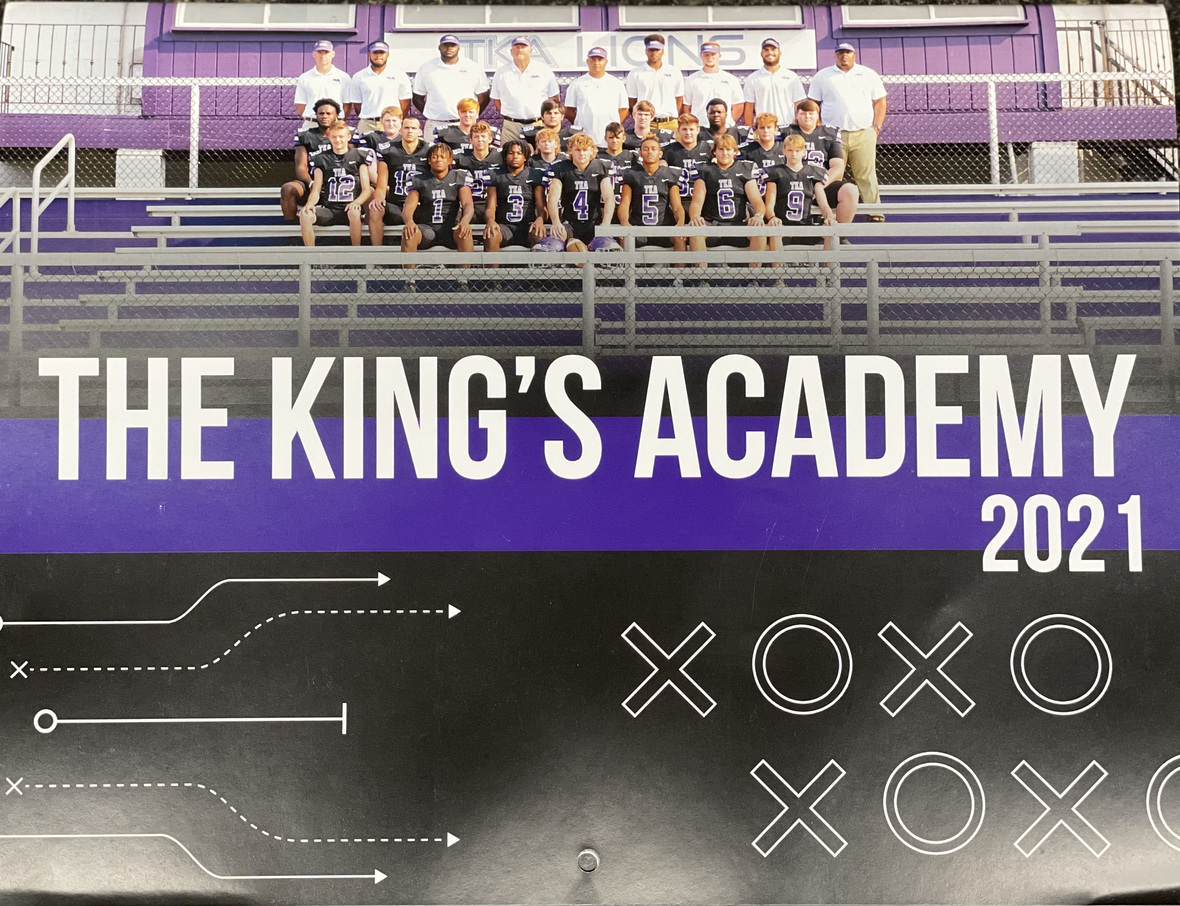# 2021-2022 Football Calendar

This year's football calendars are for sale for \$10! Stop by the school office to pick up your copy today!

 table div table+table+table+table+table+table+table+table+table+table+table+table+table+table+table+table+table+table+table+table+table+table+table+table+table+table+table+table+table+table+table+table+table+table+table+table+table+table+table div table{width:100%;padding:0}table div table+table+table+table+table+table+table+table+table+table+table+table+table+table+table+table+table+table+table+table+table+table+table+table+table+table+table+table+table+table+table+table+table+table+table+table+table+table+table div table img{width:96.23%;padding:0;float:none}table div table+table+table+table+table+table+table+table+table+table+table+table+table+table+table+table+table+table+table+table+table+table+table+table+table+table+table+table+table+table+table+table+table+table+table+table+table+table+table div table td{width:100%;padding:0 1.88% 18px}/* styles */table div table+table+table+table+table+table+table+table+table+table+table+table+table+table+table+table+table+table+table+table+table+table+table+table+table+table+table+table+table+table+table+table+table+table+table+table+table+table+table+table div table td,table.module-39{width:100%;padding:0}table div table+table+table+table+table+table+table+table+table+table+table+table+table+table+table+table+table+table+table+table+table+table+table+table+table+table+table+table+table+table+table+table+table+table+table+table+table+table+table+table div table{width:100%;float:none;margin-left:auto;margin-right:auto;padding:0}table div table+table+table+table+table+table+table+table+table+table+table+table+table+table+table+table+table+table+table+table+table+table+table+table+table+table+table+table+table+table+table+table+table+table+table+table+table+table+table+table div table a{border:0 none;text-decoration:none}table div table+table+table+table+table+table+table+table+table+table+table+table+table+table+table+table+table+table+table+table+table+table+table+table+table+table+table+table+table+table+table+table+table+table+table+table+table+table+table+table div table img{width:100%!important;border:0 none;text-decoration:none}/* styles */
 table div table+table+table+table+table+table+table+table+table+table+table+table+table+table+table+table+table+table+table+table+table+table+table+table+table+table+table+table+table+table+table+table+table+table+table+table+table+table+table+table+table div table{width:100%;padding:0}table div table+table+table+table+table+table+table+table+table+table+table+table+table+table+table+table+table+table+table+table+table+table+table+table+table+table+table+table+table+table+table+table+table+table+table+table+table+table+table+table+table div table img{width:96.23%;padding:0;float:none}table div table+table+table+table+table+table+table+table+table+table+table+table+table+table+table+table+table+table+table+table+table+table+table+table+table+table+table+table+table+table+table+table+table+table+table+table+table+table+table+table+table div table td{width:100%;padding:0 1.88% 18px}/* styles */# Covid-19 Procedures

While we are excited to offer in-person education, we must continue to maintain a safe learning environment for all students, faculty/staff, and additional stakeholders.

To do so, we will be working closely with the Sevier County Health Department in regards to Covid-19 protocols.

 table div table+table+table+table+table+table+table+table+table+table+table+table+table+table+table+table+table+table+table+table+table+table+table+table+table+table+table+table+table+table+table+table+table+table+table+table+table+table+table+table+table+table+table div table{width:100%;padding:0}table div table+table+table+table+table+table+table+table+table+table+table+table+table+table+table+table+table+table+table+table+table+table+table+table+table+table+table+table+table+table+table+table+table+table+table+table+table+table+table+table+table+table+table div table img{width:96.23%;padding:0;float:none}table div table+table+table+table+table+table+table+table+table+table+table+table+table+table+table+table+table+table+table+table+table+table+table+table+table+table+table+table+table+table+table+table+table+table+table+table+table+table+table+table+table+table+table div table td{width:100%;padding:0 1.88% 18px}/* styles */table div table+table+table+table+table+table+table+table+table+table+table+table+table+table+table+table+table+table+table+table+table+table+table+table+table+table+table+table+table+table+table+table+table+table+table+table+table+table+table+table+table+table+table+table+table div table{width:100%;padding:0}table div table+table+table+table+table+table+table+table+table+table+table+table+table+table+table+table+table+table+table+table+table+table+table+table+table+table+table+table+table+table+table+table+table+table+table+table+table+table+table+table+table+table+table+table+table div table img{width:96.23%;padding:0;float:none}table div table+table+table+table+table+table+table+table+table+table+table+table+table+table+table+table+table+table+table+table+table+table+table+table+table+table+table+table+table+table+table+table+table+table+table+table+table+table+table+table+table+table+table+table+table div table td{width:100%;padding:0 1.88% 18px}/* styles */